## Content

### Parallel, intersecting and perpendicular lines

The axioms for Euclidean geometry include: Two lines meet at a point or are parallel.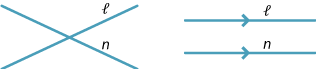Amongst those pairs of lines which meet, some are perpendicular.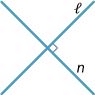If lines $$l$$ and $$n$$ are not vertical, then they are parallel if and only if they have the same gradient $$m=\tan\theta$$.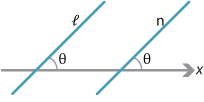Clearly, two horizontal lines are parallel. Also, any two vertical lines are parallel.

If lines $$l$$ and $$n$$ are not parallel, then their point of intersection can be found by solving the equations of the two lines simultaneously.

#### Example

Determine whether the following pairs of lines are parallel or identical and, if they are not, find the (unique) point of intersection:

1. \begin{aligned}[t] y &= x+3\\ y &= x+6 \end{aligned}
2. \begin{aligned}[t] y &= x+3\\ 3y &= 3x+7 \end{aligned}
3. \begin{aligned}[t] y &= x+3\\ 5y &= 5x+15 \end{aligned}
4. \begin{aligned}[t] y &= x+3\\ 2y &= x-2. \end{aligned}

#### Solution

1. Both lines have gradient $$m=1$$, but they have different $$y$$-intercepts. So the two lines are parallel.
2. The lines $$y=x+3$$ and $$y=x+\dfrac{7}{3}$$ have the same gradient and are therefore parallel.
3. The line $$5y=5x+15$$ can also be written as $$y=x+3$$. So the two lines are the same.
4. Number the equations: \begin{alignat*}{2} y &= x+3 &\qquad&(1)\\ 2y &= x-2. &&(2) \end{alignat*} Substituting (1) into (2) gives $$2(x+3)=x-2$$, so $$2x+6=x-2$$. Therefore $$x=-8$$ and $$y=-5$$, and the point of intersection is $$(-8,-5)$$.

Exercise 6

1. Find the equation of the line parallel to the $$x$$-axis which passes through the point where the lines $$4x+3y-6=0$$ and $$x-2y-7=0$$ meet.
2. Find the gradient of the line which passes through the point $$(2,3)$$ and the point of intersection of the lines $$3x+2y=2$$ and $$4x+3y=7$$.

Screencast of exercise 6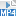#### Perpendicular lines

All vertical lines $$x=a$$ are perpendicular to all horizontal lines $$y=b$$. So, in the following, we discuss lines with equations $$y=mx+b$$, where $$m\ne0$$.

###### Theorem

The lines $$y=mx+b$$ and $$y=m_1x+c$$ are perpendicular if and only if $$mm_1=-1$$.

###### Proof

It is sufficient to prove that $$y=mx$$ is perpendicular to $$y=m_1x$$ if and only if $$mm_1=-1$$.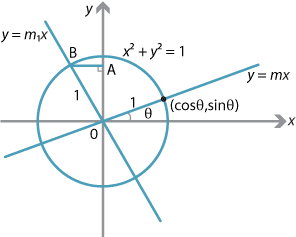Detailed description

Suppose $$y=mx$$ meets the $$x$$-axis at angle $$\theta$$, that is, $$m=\tan\theta$$. For convenience, assume that $$m>0$$. Then $$y=mx$$ meets the unit circle at $$(\cos\theta,\sin\theta)$$ in the first quadrant. If $$y=m_1x$$ is perpendicular to $$y=mx$$, then $$\angle AOB=\theta$$, $$AB=\sin\theta$$, $$OA=\cos\theta$$ and $$B$$ is $$(-\sin\theta,\cos\theta)$$. So

$m_1 = \dfrac{\cos\theta}{-\sin\theta} = -\dfrac{1}{\tan\theta} = -\dfrac{1}{m},$

as required.

Conversely, suppose $$mm_1=-1$$ and $$m=\tan\theta$$. Then

$m_1 = -\dfrac{1}{\tan\theta} = -\dfrac{\cos\theta}{\sin\theta} = \dfrac{\sin \bigl(\dfrac{\pi}{2}+ \theta\bigr)}{\cos\bigl(\dfrac{\pi}{2}+\theta \bigr)} = \tan\Bigl(\dfrac{\pi}{2}+\theta\Bigr).$

Hence $$y=mx$$ is perpendicular to $$y=m_1x$$.

$$\Box$$

This result is often stated as:

The line $$y=m_1x+c_1$$ is perpendicular to the line $$y=m_2x+c_2$$ if and only if $$m_1m_2=-1$$.

Exercise 7

Use the angle sum formulas

\begin{align*} \sin(A+B) &= \sin A\,\cos B+\cos A\,\sin B\\ \cos(A+B) &= \cos A\,\cos B-\sin A\,\sin B \end{align*} to show that $\tan(90^\circ+\theta) = -\dfrac{1}{\tan\theta}.$

Then use this identity to give an alternative proof that lines $$y=mx$$ and $$y=m_1x$$ are perpendicular if and only if $$mm_1=-1$$.

#### Example

Find the equation of the line $$l$$ through $$(1,3)$$ perpendicular to the line $$2x+3y=12$$. Find the equation of the line through $$(4,5)$$ parallel to $$l$$.

#### Solution

The gradient of the line $$2x+3y=12$$ is $$-\dfrac{2}{3}$$, so $$l$$ has gradient $$\dfrac{3}{2}$$. The equation of $$l$$ is

\begin{align*} y-3 &= \dfrac{3}{2}(x-1)\\ 2y-6 &= 3x-3\\ 3x-2y+3 &= 0. \end{align*}

The other line has equation $$y-5=\dfrac{3}{2}(x-4)$$ or, equivalently, $$3x-2y-2=0$$.

#### Example

For each pair of lines, determine whether they are parallel, identical or meet. If they meet, find the point of intersection and whether they are perpendicular.

1. $$l:\ 3x=2y+5,\quad n:\ 2x+3y=7$$
2. $$l:\ 6x=5y+7,\quad n:\ 12x=10y+13$$
3. $$l:\ 3y=2x-1,\quad n:\ 4x=5y-7$$
4. $$l:\ 5y=2x+2,\quad n:\ 15y-6x-6=0$$

#### Solution

1. The gradient of $$l$$ is $$\dfrac{3}{2}$$ and the gradient of $$n$$ is $$-\dfrac{2}{3}$$. Since $\dfrac{3}{2}\times -\dfrac{2}{3} = -1,$ the lines are perpendicular.
We have \begin{alignat*}{2} 6x &= 4y+10 &\qquad&\text{from $$l$$,}\\ 6x+9y &= 21 &&\text{from $$n$$.} \end{alignat*} Substituting, we obtain $$13y+10=21$$ and so $y=\dfrac{11}{13}, \qquad x=\dfrac{29}{13}.$ The point of intersection is $$\Bigl(\dfrac{29}{13},\dfrac{11}{13}\Bigr)$$.
2. The lines $$l$$ and $$n$$ have the same gradient $$\dfrac{6}{5}$$. The line $$l$$ has $$x$$-intercept $$\dfrac{7}{6}$$, and the line $$n$$ has $$x$$-intercept $$\dfrac{13}{12}$$. So $$l$$ is parallel to $$n$$, but $$l\ne n$$.
3. The gradient of $$l$$ is $$\dfrac{2}{3}$$ and the gradient of $$n$$ is $$\dfrac{4}{5}$$. So $$l$$ meets $$n$$, but $$l$$ is not perpendicular to $$n$$.

We have
\begin{alignat*}{2} 15y &= 10x-5 &\qquad&\text{from $$l$$,}\\ 12x &= 15y-21 &&\text{from $$n$$.} \end{alignat*} Substituting, we obtain $$12x=10x-5-21$$. So $$x=-13$$ and $$y=-9$$. The point of intersection is $$(-13,-9)$$.
4. Rearranging $$15y-6x-6=0$$ gives $$5y=2x+2$$. So $$l$$ and $$n$$ are the same line.

Exercise 8

Consider the line $$l$$ with equation $$ax+by+c=0$$ and the point $$P(x_1,y_1)$$.

1. Show that the line through $$P$$ parallel to $$l$$ is given by $$ax+by=ax_1+by_1$$.
2. Show that the line through $$P$$ perpendicular to $$l$$ is given by $$bx-ay=bx_1-ay_1$$.

Next page - Content - Perpendicular distances